Test: Laplace Transform- 1

# Test: Laplace Transform- 1

Test Description

## 10 Questions MCQ Test Signals and Systems | Test: Laplace Transform- 1

Test: Laplace Transform- 1 for Electronics and Communication Engineering (ECE) 2023 is part of Signals and Systems preparation. The Test: Laplace Transform- 1 questions and answers have been prepared according to the Electronics and Communication Engineering (ECE) exam syllabus.The Test: Laplace Transform- 1 MCQs are made for Electronics and Communication Engineering (ECE) 2023 Exam. Find important definitions, questions, notes, meanings, examples, exercises, MCQs and online tests for Test: Laplace Transform- 1 below.
Solutions of Test: Laplace Transform- 1 questions in English are available as part of our Signals and Systems for Electronics and Communication Engineering (ECE) & Test: Laplace Transform- 1 solutions in Hindi for Signals and Systems course. Download more important topics, notes, lectures and mock test series for Electronics and Communication Engineering (ECE) Exam by signing up for free. Attempt Test: Laplace Transform- 1 | 10 questions in 30 minutes | Mock test for Electronics and Communication Engineering (ECE) preparation | Free important questions MCQ to study Signals and Systems for Electronics and Communication Engineering (ECE) Exam | Download free PDF with solutions
 1 Crore+ students have signed up on EduRev. Have you?
Test: Laplace Transform- 1 - Question 1

### Determine the Laplace transform of given signal. Q. x(t) = u( t - 2)

Detailed Solution for Test: Laplace Transform- 1 - Question 1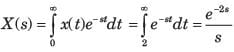Test: Laplace Transform- 1 - Question 2

### Laplace transform of t2 sin⁡(2t).

Detailed Solution for Test: Laplace Transform- 1 - Question 2

We know that,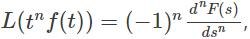Here, f(t) = sin⁡(2t) => F(s) =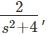Hence,  L(t2sin(2t)) =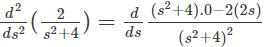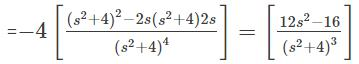Test: Laplace Transform- 1 - Question 3

### Determine the Laplace transform of given signal. Q. x(t) = e-2tu(t+1)

Detailed Solution for Test: Laplace Transform- 1 - Question 3

Laplace transform e-at u(t) = 1/s+a

for all Re(s) > - Re(a)

e-2t u(t+1) = 1/(s+2)

Test: Laplace Transform- 1 - Question 4

Determine the Laplace transform of given signal.
Q. x(t) = e2tu(-t+2)

Detailed Solution for Test: Laplace Transform- 1 - Question 4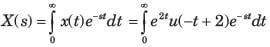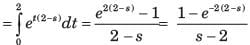Test: Laplace Transform- 1 - Question 5

Determine the Laplace transform of given signal.
Q. x(t) = sin 5 t

Detailed Solution for Test: Laplace Transform- 1 - Question 5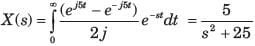Test: Laplace Transform- 1 - Question 6

Determine the Laplace transform of given signal.
Q. x( t) = u(t) - u(t - 2)

Detailed Solution for Test: Laplace Transform- 1 - Question 6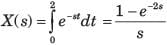Test: Laplace Transform- 1 - Question 7

Determine the Laplace transform of given signal.
Q. x( t) = d/dt {te-1u(t)}

Detailed Solution for Test: Laplace Transform- 1 - Question 7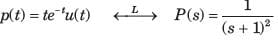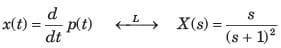Test: Laplace Transform- 1 - Question 8

Determine the Laplace transform of given signal.
Q. x( t) = tu(t)*cos2πt u(t)

Detailed Solution for Test: Laplace Transform- 1 - Question 8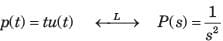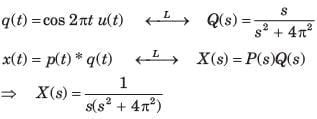Test: Laplace Transform- 1 - Question 9

Determine the Laplace transform of given signal.
Q. x( t) = t3u(t)

Detailed Solution for Test: Laplace Transform- 1 - Question 9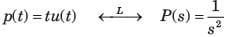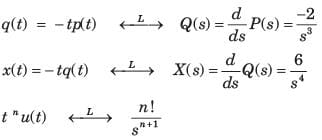Test: Laplace Transform- 1 - Question 10

Determine the Laplace transform of given signal.
Q. x(t) = u( t - 1) * e-2tu( t - 1)

Detailed Solution for Test: Laplace Transform- 1 - Question 10

L{−e-at u(t)}

= ∫(∞ to −∞) −e-at u(t)e-stdt

L{−e-at u(t)}

= ∫(∞ to 0) − e-(s+a)t dt

= - |e-(s+a)t/−(s+a)|∞ to 0

= −1/s+a

=> L{−e-2t u(t)} = -1/s+2

=> L{−e-2t u(t-1)} = e-(s+2)/s2 …………(1)

q(t) = L{u(t-1)}

= e-s/(s2)................(2)

Multiply (1) and (2)

= e-2(s+1)/(s+2)

## Signals and Systems

40 videos|44 docs|33 tests
 Use Code STAYHOME200 and get INR 200 additional OFF Use Coupon Code
Information about Test: Laplace Transform- 1 Page
In this test you can find the Exam questions for Test: Laplace Transform- 1 solved & explained in the simplest way possible. Besides giving Questions and answers for Test: Laplace Transform- 1, EduRev gives you an ample number of Online tests for practice

## Signals and Systems

40 videos|44 docs|33 tests